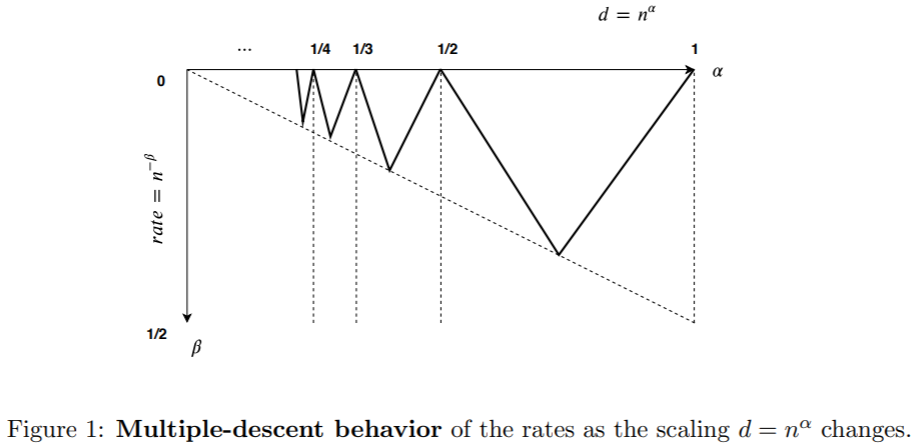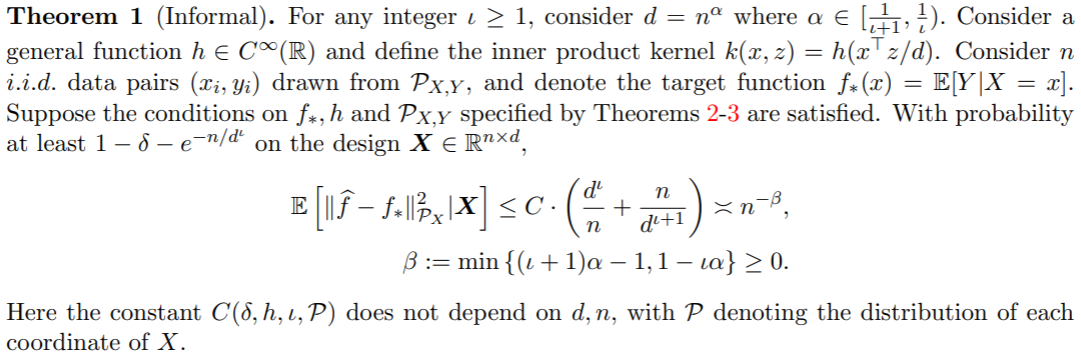# Multiple Descent of Minimum-Norm Interpolants

##### Posted on Oct 11, 20210 Comments

The paper investigate the generalization and consistency of minimum-norm interpolants

$\hat f\in \argmin_{f\in \cH} \Vert f\Vert_{\cal H}\quad \st \quad f(x_i) = y_i,i=1,\ldots,n$

of the data $(x_1,y_1),\ldots, (x_n,y_n)\in \IR^d\times \IR$ w.r.t. a norm in a RKHS $\cH$.

The interpolant is also called Kernel Ridgeless Regression, can be viewed as a limiting solution of

$\argmin_{f\in\cH} \frac 1n \sum_{i=1}^n (f(x_i)-y_i)^2 + \lambda \Vert f\Vert_\cH^2$

as $\lambda\rightarrow 0$.

Recent literature has focused on understanding risk of estimators that interpolate data, including

• Belkin et al. (2018): nonparametric local rules
• Belkin et al. (2019) & Hastie et al. (2019): high-dimensional linear regression
• Ghorbani et al. (2019): random features model
• Feldman (2019): classification with rare instances
• Belkin et al. (2018), Liang and Rakhlin (2018), Rakhlin and Zhai (2018): kernel (ridgeless) regression
• Rakhlin and Zhai (2018): the minimum-norm interpolant w.r.t. Laplace kernel is not consistent if dimensionality $d$ of the data is constant w.r.t. $n$
• Liang and Rakhlin (2018): when $n \asymp d$, risk can be upper bounded by quantity that can be small under favorable spectral properties of the data and the kernel matrix.

The paper studies the performance of the minimum-norm interpolants in a general scaling regime $d\asymp n^\alpha, \alpha\in (0, 1)$.

Non-monotone behavior of the upper bound on the risk of the minimum-norm interpolant,the interpolating solution provably has a diminishing (in sample size) out-of-sample error for most of the scalings of $d$ and $n$Published in categories Note